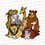# Elementary Techniques used in the IMO (International Mathematical Olympiad) - Cauchy Schwarz Inequality

Continuing from: Part 1

Part 2

Applications:

Example 1: (Iran 1998) Suppose that $x,y,z \geq 1$ and $\frac{1}{x} + \frac{1}{y} + \frac{1}{z} = 2$. Prove that:

$\sqrt{x+y+z} \geq \sqrt{x-1} + \sqrt{y-1} + \sqrt{z-1}$.

Solution: The condition given is considerably weird. In a sense, we would like to involve the variables more than just on the denominators. We have $2$ approaches, either use a substitution or try to rewrite it in a nicer form. It seems that the latter works. We notice that most conditions have the constant given as $1$ so it is pretty motivated to write the hypothesis as:

$\frac{x-1}{x} + \frac{y-1}{y} + \frac{z-1}{z} = 1$.

Now notice how "magically" we have $(x-1), (y-1), \ldots$ on the numerator. In fact, due to the fact that we can square both sides, we can immediately see how to apply Cauchy:

$\sum_{cyc}x = ( \sum_{cyc}x)( \sum_{cyc} \frac{x-1}{x}) \geq ( \sum_{cyc} \sqrt{x-1} )^2$. ■

Example 2: (Japan 2004) Let $a,b,c$ be positive real numbers with sum $1$. Prove that

$\frac{1+a}{1-a} + \frac{1+b}{1-b} + \frac{1+c}{1-c} \leq \frac{2a}{b} + \frac{2b}{c} + \frac{2c}{a}$.

Solution: With a little experience in inequalities, we see some use for $a + b + c = 1$:

1. Substitute into the question

2. Substitue as $a = \frac{x}{x+y+z}, b = \frac{y}{x+y+z}, etc$

Now, also note that we do NOT want to make the inequality in question more complicated, so we opt to using the former. Also with some experience we see that we do not like fractions such as $\frac{1+a}{1-a}$ or $\frac{1-a}{1+a}$ for the matter. We like to only have the variable and no constants (or vice versa) on the denominator so that we can easily form squares. Therefore, by substituting and dividing both sides by $2$ to make the RHS $\frac{a}{b} , \ldots$, we rewrite the inequality as:

$\frac{3}{2} + \frac{a}{b+c} + \frac{b}{a+b} + \frac{c}{a+b} \leq \frac{a}{b} + \frac{b}{c} + \frac{c}{a}$

We isolate the constant and rearrange to get:

$\sum_{cyc} \frac{ac}{b(b+c)} \geq \frac{3}{2}$

Intuitively, we multiply both numerator and denominator of the LHS by $ac$ (taken cyclically), and directly apply Cauchy Schwarz,

$\sum_{cyc} \frac{ac}{b(b+c)} = \sum_{cyc} \frac{a^2c^2}{abc(a+c)} \geq \frac{(ab+bc+ca)^2}{2abc(a+b+c)} \geq \frac{3}{2}$

Example 3: (IMO SL 2011) Given arbitrary real numbers $x_1, x_2, \ldots, x_n$, prove that:

$\frac{x_1}{1+x_1^2} + \frac{x_2}{1+x_1^2+x_2^2} + \ldots + \frac{x_n}{1+x_1^2 + x_2^2 + \ldots + x_n^2}$.

Solution: We recall the extremely useful variant of Cauchy:

$n(a_1^2 + a_2^2 + \ldots + a_n^2) \geq (a_1 + a_2 + \ldots + a_n)^2$

So, what we do is that, we apply the above inequality directly to the given inequality in question. The motivation behind this step is to generate an $n$ term. So,

$(\frac{x_1}{1+x_1^2} + \frac{x_2}{1+x_1^2 + x_2^2} + \ldots + \frac{x_n}{1+x_1^2 + x_2^2 + \ldots + x_n^2})^2 \leq [(\frac{x_1}{1+x_1^2})^2 + \ldots + (\frac{x_n}{1+x_1^2+x_2^2 + \ldots + x_n^2})^2] \times n$.

Notice also that for $k \geq 2$, we have:

$(\frac{x_k}{1+x_1^2+x_2^2 + \ldots + x_k^2})^2 = \frac{x_k^2}{1+x_1^2+x_2^2 + \ldots + x_k^2)^2}$

$\leq \frac{x_k^2}{(1+x_1^2+x_2^2 + \ldots + x_k^2)(1+x_1^2+x_2^2 + \ldots + x_{k-1}^2)}$

$\leq \frac{1}{1+x_1^2+x_2^2 + \ldots + x_{k-1}^2} - \frac{1}{1+x_1^2+x_2^2 + \ldots + x_k^2}$.

The idea here is that in order to generate $n$ we might as well try to eliminate the variables by telescoping. Observe finally, that for $k = 1$, we have:

$(\frac{x_1}{1+x_1^2})^2 \leq \frac{x_1^2}{1+x_1^2} = 1 - \frac{1}{1+x_1^2}$

I leave my reader to finish the argument.■

These daily instalments will be paused until Friday afternoon, Singapore time because I am going overseas.Note by Anqi Li
6 years, 11 months ago

This discussion board is a place to discuss our Daily Challenges and the math and science related to those challenges. Explanations are more than just a solution — they should explain the steps and thinking strategies that you used to obtain the solution. Comments should further the discussion of math and science.

When posting on Brilliant:

• Use the emojis to react to an explanation, whether you're congratulating a job well done , or just really confused .
• Ask specific questions about the challenge or the steps in somebody's explanation. Well-posed questions can add a lot to the discussion, but posting "I don't understand!" doesn't help anyone.
• Try to contribute something new to the discussion, whether it is an extension, generalization or other idea related to the challenge.

MarkdownAppears as
*italics* or _italics_ italics
**bold** or __bold__ bold
- bulleted- list
• bulleted
• list
1. numbered2. list
1. numbered
2. list
Note: you must add a full line of space before and after lists for them to show up correctly
paragraph 1paragraph 2

paragraph 1

paragraph 2

[example link](https://brilliant.org)example link
> This is a quote
This is a quote
    # I indented these lines
# 4 spaces, and now they show
# up as a code block.

print "hello world"
# I indented these lines
# 4 spaces, and now they show
# up as a code block.

print "hello world"
MathAppears as
Remember to wrap math in $$ ... $$ or $ ... $ to ensure proper formatting.
2 \times 3 $2 \times 3$
2^{34} $2^{34}$
a_{i-1} $a_{i-1}$
\frac{2}{3} $\frac{2}{3}$
\sqrt{2} $\sqrt{2}$
\sum_{i=1}^3 $\sum_{i=1}^3$
\sin \theta $\sin \theta$
\boxed{123} $\boxed{123}$

Sort by:

Great post! and the main thing is you are involving motivation to every problem..Good job Anqi!

- 6 years, 11 months ago

- 6 years, 11 months ago

Nice my friend.

- 6 years, 11 months ago

easy

- 6 years, 11 months ago

can you give me refence for this material ??

- 6 years, 11 months ago

- 6 years, 5 months ago

Anqi Li Can you add this to the Cauchy Schwarz Inequality Wiki page?

Staff - 6 years, 1 month ago